# 【自学】深度学习入门 基于python的理论与实现 LESSON4 ＜神经网络的学习1＞

Rachel MuZy · 收录于 2023-11-21 05:05:19 · source URL

1. 均方误差

2. 交叉熵误差

3. mini-batch学习

4. mini-batch版交叉熵误差的实现

（1）t 为one-hot形式的交叉熵误差

（2）t 为非one-hot形式的交叉熵误差

5. 为何要设定损失函数

## 前言

这里的“学习”是指从训练数据中自动获取最优权重参数的过程。学习的目的就是以损失函数为基准，找出能使它的值达到最小的权重参数。为了找出尽可能小的损失函数的值，本节我们利用函数斜率的梯度法。

## 一、损失函数

1. 神经网络以某个指标为线索寻找最优权重参数，该指标称为损失函数，一般用均方误差和交叉熵误差等。

2. 损失函数是表示神经网络性能的“恶劣程度”指标，即当前的神经网络对监督数据在多大程度上不拟合。

### 1. 均方误差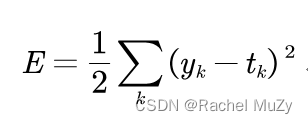式中，yk表示神经网络的输出，tk表示监督数据，k表示数据的维数。

``````import numpy as np

def MSE(y, t):
square = (y - t) * (y - t)
E = 0.5 * np.sum(square, axis = 1)
return E

y = np.array([[0.1, 0.05, 0.6, 0.0, 0.05, 0.1, 0.0, 0.1, 0.0, 0.0], [0.1, 0.05, 0.6, 0.0, 0.05, 0.1, 0.0, 0.1, 0.0, 0.0]])
t = np.array([[0, 0, 1, 0, 0, 0, 0, 0, 0, 0], [0, 0, 1, 0, 0, 0, 0, 0, 0, 0]])
output = MSE(y, t)
print(output)``````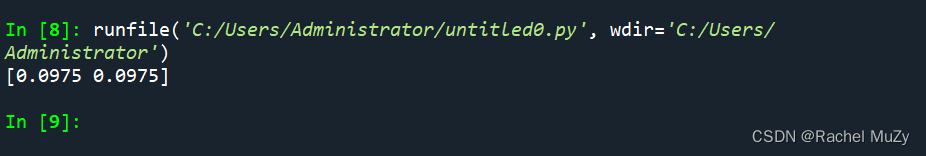### 2. 交叉熵误差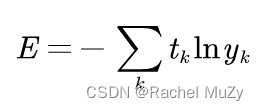式中，log表示以为底数的自然对数，yk是神经网络的输出，tk是正确解的标签。并且tk中只有正确解标签的索引为1，其他均为0。

``````import numpy as np

def cross_entropy_error(y, t):
delta = 1e-7
E = -np.sum(t * np.log(y + delta), axis = 1)
return E

y = np.array([[0.1, 0.05, 0.6, 0.0, 0.05, 0.1, 0.0, 0.1, 0.0, 0.0], [0.1, 0.05, 0.1, 0.0, 0.05, 0.1, 0.0, 0.6, 0.0, 0.0]])
t = np.array([[0, 0, 1, 0, 0, 0, 0, 0, 0, 0], [0, 0, 1, 0, 0, 0, 0, 0, 0, 0]])
output = cross_entropy_error(y, t)
print(output)``````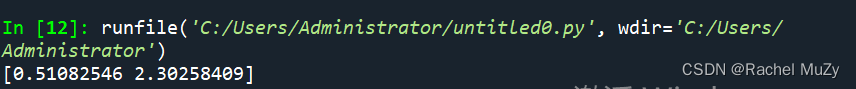### 3. mini-batch学习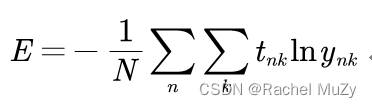``````import numpy as np

def dimension(y):
line = len(y)
return line

def cross_entropy_error(y, t, line):
delta = 1e-7
E_pro = np.sum(t * np.log(y + delta), axis = 1)
E = -np.sum(E_pro, axis = 0) / line
return E

y = np.array([[0.1, 0.05, 0.6, 0.0, 0.05, 0.1, 0.0, 0.1, 0.0, 0.0], [0.1, 0.05, 0.1, 0.0, 0.05, 0.1, 0.0, 0.6, 0.0, 0.0]])
t = np.array([[0, 0, 1, 0, 0, 0, 0, 0, 0, 0], [0, 0, 1, 0, 0, 0, 0, 0, 0, 0]])
line = dimension(y)
output = cross_entropy_error(y, t, line)
print(output)``````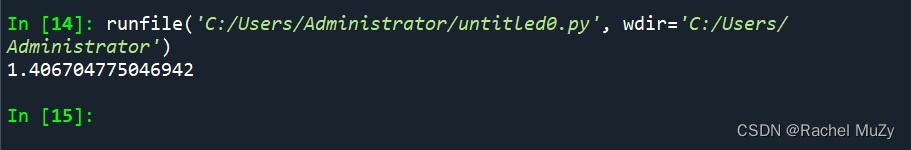### 4. mini-batch版交叉熵误差的实现

#### （1）t 为one-hot形式的交叉熵误差

``````import numpy as np

def cross_entropy_error(y, t):
if y.ndim == 1:
t = t.reshape(1, t.size)
y = y.reshape(1, y.size)

batch_size = y.shape
return -np.sum(t * np.log(y + 1e-7)) / batch_size

y = np.array([[0.1, 0.05, 0.6, 0.0, 0.05, 0.1, 0.0, 0.1, 0.0, 0.0], [0.1, 0.05, 0.1, 0.0, 0.05, 0.1, 0.0, 0.6, 0.0, 0.0]])
t = np.array([[0, 0, 1, 0, 0, 0, 0, 0, 0, 0], [0, 0, 1, 0, 0, 0, 0, 0, 0, 0]])
output = cross_entropy_error(y, t)
print(output)``````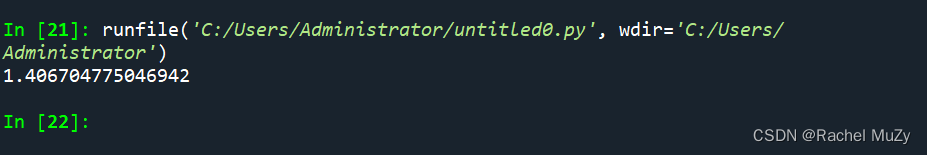（1）由于one-hot表示中t为0的元素的交叉熵误差也为0，因此针对这些元素的计算可以忽略。换言之，如果可以获得神经网络在正确解标签处的输出，就可以计算交叉熵误差。因此t为one-hot表示时通过t * np.log(y)计算。

（2）ndim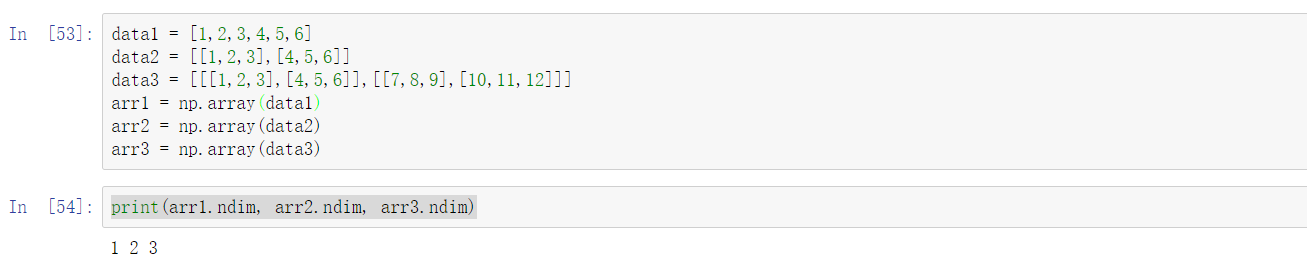ndim返回的是数组的维度，返回的只有一个数，该数即表示数组的维度。

（3）shape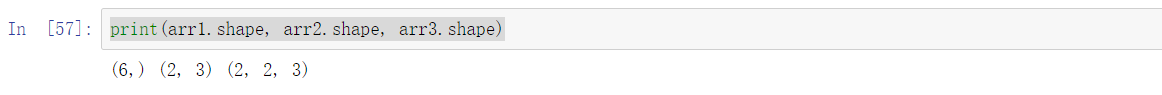shape：表示各位维度大小的元组。返回的是一个元组。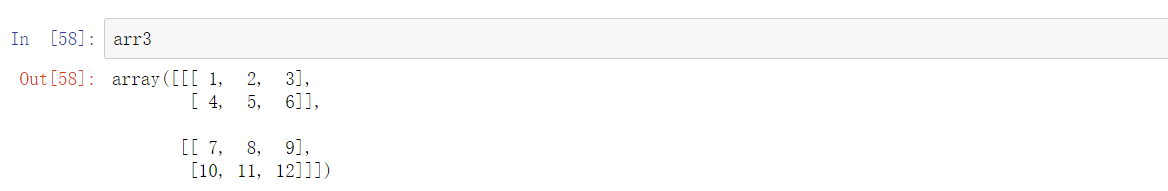#### （2）t 为非one-hot形式的交叉熵误差

``````import numpy as np

def cross_entropy_error(y, t):
if y.ndim == 1:
t = t.reshape(1, t.size)
y = y.reshape(1, y.size)

batch_size = y.shape
return -np.sum(np.log(y[np.arange(batch_size), t] + 1e-7) / batch_size``````

## 二、数值微分

（1）梯度法使用梯度的信息决定前进的方向，下一节将介绍梯度是什么、有什么性质等内容。本节先介绍导数。

（2）利用微小的差分求导的过程称为数值微分，下面举一个数值微分的例子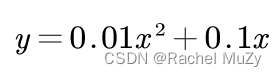代码实现：

``````from sympy import diff
from sympy import symbols
import numpy as np
import matplotlib.pylab as plt

def function_1(x):
y = 0.01 * x ** 2 + 0.1 * x
return y

x = np.arange(0.0, 20.0, 0.1)
y1 = function_1(x)
plt.plot(x, y1)
plt.show()

x = symbols("x")
d = diff(function_1(x),x)
y2 = np.array(d)
plt.plot(x, y2)
plt.show``````